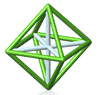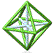Park City Mathematics Institute Secondary School Teacher Program Reflection on Practice Class: Day 5 Akihiko Takahashi

Aki: I walked around looking at your posters. I want to ask each group to report.

What did you discuss at the end of yesterday's class?

Group A: Like most groups we generated a variety of methods to derive the proof of the area of a trapezoid. In going through each of these we started to discover. As we went through these it was hard to see... one of the things we seemed to discover. This type of activity could be used in a 5th grade, 9th grade, 11th grade classroom depending on level of depth. So you could decide what type of questions to ask.

Aki: Consider when you slide the top base of trapezoid to make a rectangle or parallelogram. Does sliding change the area? Is that appropriate for a 5th grade classroom?

Group B: There was a lot of variety of solutions. Even though each table had a bunch, the other tables presented ones we hadn't thought of. Some solutions were grouped by solution method. Others categorized solutions based on immediate previous knowledge. for example areas of parallelograms or whether they were based on much earlier techniques. There were different ways of looking at how to group the answers and how to move through these to help students develop a global and more sophisticated level of understanding.

Group C: I would estimate that we went through a very similar process. What was interesting when we were trying to pick the 3 or 4 models to present to the whole group - we had a lot of ideas of which ones were the clearest or which would take the kids where we want them to go. The experiences we were having as teachers mirrored what the kids would go through themselves. We need to remember that flexibility.

Aki: Some methods generalize. Others don't.

One method involved extending the non-parallel sides to make a large triangle. Big one = .5*b1(h + x). The other triangle = .5*b2*x. So we subtract to get the area of the trap.

= .5*b1(h+x)-.4*b2*x

You can't get rid of the x's algebraically, but if you notice the triangles are similar you can get the desired formula. h/(h+x) = B2/B1

Should we categorize? Or should the students categorize?

Aki: Deriving the formula and finding the area are different. Some students find the area via counting - but that doesn't generalize to finding the formula.

Question 3) How to facilitate discussion. How can students really appreciate someone else's method? What kind of ideas? Of course there are many.

We start with having them recognize what area is from their experiences of rectangle and triangle - to help them find area of trapezoid.

We can remind them when we were doing the previous problem of parallelogram and triangle we had done them by putting things together or taking them apart. Maybe we could invoke what we've done before in a slightly different way to get at this.

Or we could simply transform this shape into another shape. (Draw line from vertex through midpoint of opposite side to reflect triangle part to make a triangle.)

3 basic methods - add things to diagram.
Take things away
Transform

Which might be easy for students to figure out?

Group D: We had some methods that didn't generalize. That might be interesting for students to make a trapezoid where a particular method doesn't work.

To teach math, we need to look at all of these possibilities.

Help students develop problem solving strategies.

1. Minimize the number of cuts.
2. List available previous knowledge that might be able to help derive the formula.

Better to use rectangle > parallelogram > triangle > to find area of trapezoid

How can we save our effort? Which method might be more efficient? Students have already known that triangles with same base and same height have same area.

Draw line parallel from one vertex parallel to diagonal of trap. Use property that students know: that triangles with the same base and same height have same area.

Aki: If you really expect students to think, you should be very, very mean. The process is better when you use rectangle > parallelogram > triangle > to find area of trapezoid. This process is very important for students - as it relates to their progress in future math

Note: The formula for trapezoid works for parallelogram, rectangle, and square.

Can we generalize the area of a trapezoid method this to arbitrary quadrilaterals? Yes - divide it into two triangles (whose height isn't necessarily the same)

Aki then introduced Monday's problem.

Back to: Class NotesPCMI@MathForum Home || IAS/PCMI Home© 2001 - 2018 Park City Mathematics Institute IAS/Park City Mathematics Institute is an outreach program of the Institute for Advanced Study, 1 Einstein Drive, Princeton, NJ 08540 Send questions or comments to: Suzanne Alejandre and Jim King

With program support provided by Math for America

This material is based upon work supported by the National Science Foundation under DMS-0940733 and DMS-1441467. Any opinions, findings, and conclusions or recommendations expressed in this material are those of the author(s) and do not necessarily reflect the views of the National Science Foundation.